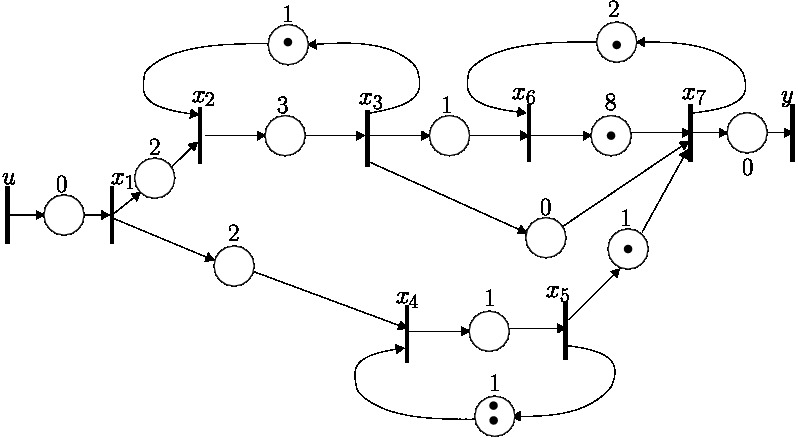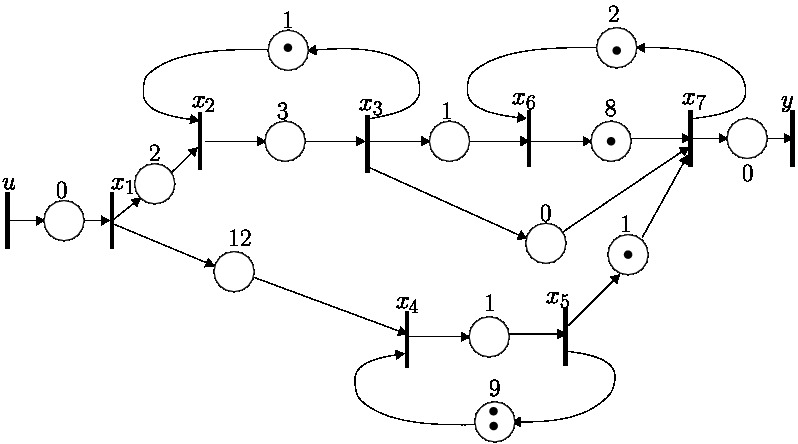Holding Time Maximization Preserving Output Perormance for Timed Event Graphs

The considered TEG is represented on the figure below:The state-space model of the previous TEG in Maxin[[γ,δ]] is:

A=

 | ε ε ε ε ε ε ε | | δ2 ε γδ ε ε ε ε | | ε δ3 ε ε ε ε ε | | δ2 ε ε ε γ2δ ε ε | | ε ε ε δ ε ε ε | | ε ε δ ε ε ε γδ2 | | ε ε e ε γδ γδ8 ε |

B=

 | e | | ε | | ε | | ε | | ε | | ε | | ε |

C=

 | ε ε ε ε ε ε e |

After holding time maximization, the modified TEG is represented on the figure below:The state-matrix Aopt of the modfied system is:

Aopt = PrγA(CA*\CA*) =

 | ε ε ε ε ε ε ε | | δ2 ε γδ ε ε ε ε | | ε δ3 ε ε ε ε ε | | δ12 ε ε ε γ2δ9 ε ε | | ε ε ε δ ε ε ε | | ε ε δ ε ε ε γδ2 | | ε ε e ε γδ γδ8 ε |

Code Source of the example considered :

The software is the library Minmaxgd extended with the γ-projection along a series.

The following file contains the extended library and the implementation of the examples of the paper: HolTMax.zip

Original library is available here : Minmaxgd

Date : December 15th, 2012